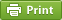Werner's Blog — Opinion, Analysis, Commentary
Electricity Economics and Demand Side Management

Building new power plants is expensive. Electric utilities calculate that paying households for conserving energy may be cheaper. Enter the ides of demand-side management (DSM)—programs that encourage consumers to modify their level and pattern of electricity usage. Originally, the emphasis of DSM programs was on deferring the need for more generating facilities and transmission capacity. DSM has grown beyond that by also focusing on enhancing customer service and providing real-time information to consumers about their electricity use. There are three broad approaches to DSM:

• Peak-load pricing is meant to shift demand from peak periods to off-peak periods. Electricity use follows seasonal and diurnal patterns. Electric utilities must provide sufficient excess capacity to meet demand at all times. Smoothing the seasonal and diurnal patterns allows utilities to reduce this excess capacity, or avoid building new power plants. In Ontario, time-of-day pricing encourages consumers to reduce electricity consumption during peak periods (mid-day in the summer, morning and evening in the winter).
• Incentive programs aim at improving energy efficiency. Such programs often involve rebates and other financial incentives to replace older energy-efficient equipment with newer more energy-efficient equipment. Replacing incandescent light bulbs with LED lights is one example. Another is subsidizing appliances that are Energy-star rated.
• Real-time metering provides instant feedback to consumers and allows them to monitor the electricity use of their homes. The idea is that better information will prompt homeowners to modify their behaviour, such as turning down thermostats, when they see the real-time cost of their behaviour. Other types of information programs tell consumers how their electricity use compares with that of similar neighbours, prompting those with excessive consumption to investigate how they could reduce their electricity bill.

In today's blog I focus on the economics of incentive programs by developing a simple model of "optimal DSM". How much should an electric utility pay to promote energy conservation and greater energy efficiency? It turns out that the answer depends crucially on quantifying two parameters.

An electric utility faces the decision to spend an amount $$r$$ to reduce electricity consumption. Demand for electricity is $$q(r)$$ so that $$q'(r)<0$$. We define the DSM elasticity $\eta\equiv \frac{\mathrm{d} q(r)}{\mathrm{d}r}\cdot\frac{r}{q} <0$ which tends to be a small negative number. Spending an amount $$r$$ helps reduce electricity consumption by fostering energy efficiency. The utility's profit function is given by $\pi=p\cdot q(r)-c(q(r))-r$ where $$p$$ is the retail price mandated by the regulator which ensures that $$\pi=0$$ and therefore $$p\cdot q=c+r$$. The profit function shows that the utility can only influence the revenue by dampening demand through paying for energy conservation measures. The cost side depends entirely on demand. As demand rises, the utility has to purchase more costly electricity (either by using its own peak-load generators or buying it in the wholesale electricity market from other companies). The first-order condition for profit maximization is $p\cdot q'(r) = c'(q)\cdot r'(q) +1$ We also introduce the cost function elasticity $\theta\equiv \frac{\mathrm{d} c(q)}{\mathrm{d}q}\cdot\frac{q}{c}>1$ and we express the DSM expenditure as a share of total cost: $$\phi\equiv r/c$$. The convexity of the cost function reflects the ordering of production assets, from low-cost base-load plants to high-cost peak-load plants. These plants do not have to be operated by the utility itself. If demand exceeds the utility's own generation assets, it has to start purchasing electricity in the wholesale market at increasing cost. After replacing the derivatives in the first-order condition with elasticities and using the zero-profit constraint to set $$p=(c+r)/q$$, rearranging the first-order condition yields $\phi\equiv \frac{r}{c} = (\theta-1)\left[\frac{\eta}{\eta-1}\right]$ The optimal ratio of DSM expenditures increases along with the convexity of the cost function and the magnitude of the DSM elasticity. The more convex the cost function, the more the utility is worried about buying extra electricity in the wholesale market. And the more responsive the DSM policies, the more the utility wants to spend on them. Note that the term in square bracket is positive when $$\eta$$ is negative. For an $$\eta$$ small in magnitude, the expression in square brackets is actually quite close to $$|\eta|$$, the absolute value of the elasticity.

Perhaps it is useful to illustrate the above condition for "optimal DSM". Let us assume that $$\eta=-0.05$$ is quite small: a 10% increase in energy efficiency incentives reduces demand by only 0.5%. Let us also assume that $$\theta=3$$, which means that the cost function is quite convex. Then the utility should spend about 9.5% of its cost on energy efficiency. That is not a small number. The convexity parameter's effect is crucial. An elasticity of 3 means that an increase in demand by 10% drives up electricity generation costs by 30% at the margin. That is not implausible when utilities have to switch to peak-load capacity or import electricity at much higher cost from neighbouring utilities. The situation is rather different when marginal costs are nearly constant. When marginal costs are exactly constant, $$\theta=1$$, and the utility does not need to worry about energy conservation, or pay consumers to reduce demand. The bottom line is simple: the closer a utility is to its capacity constraint, the more it pays to pursue DSM.

Posted on Saturday, November 7, 2015 at 07:45 — #Energy | #EconomicsRecent Blog Entries

Topics

Months

Subscribe to RSS feed© 2023  Prof. Werner Antweiler, University of British Columbia.